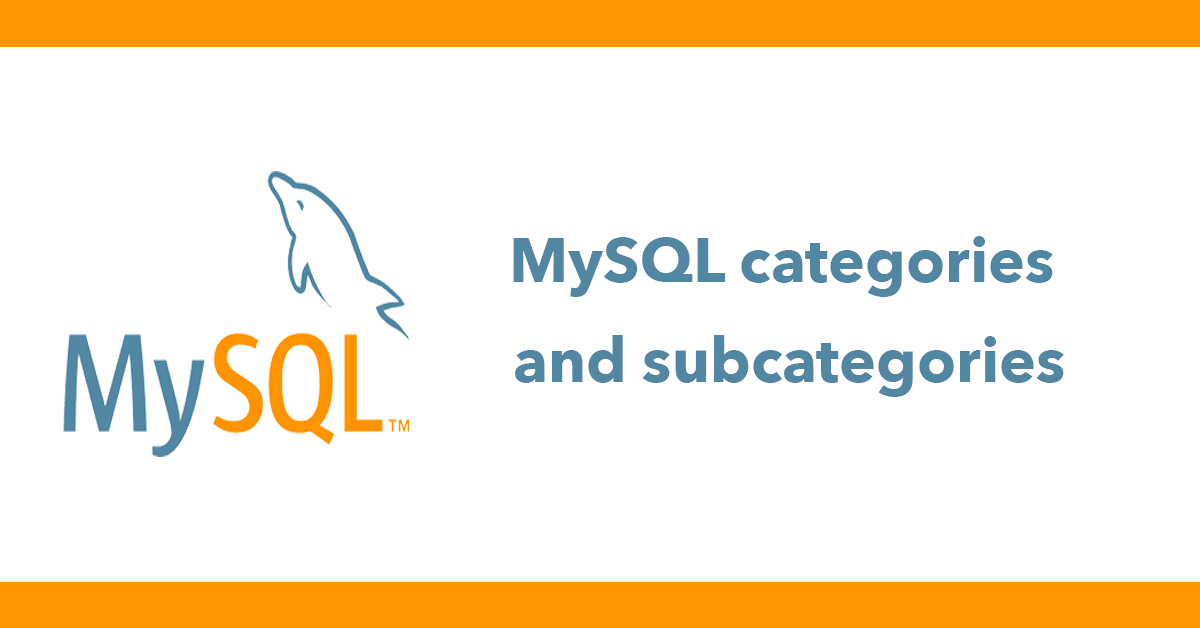# MySQL categories and subcategories

Working with categories is a common task, also is the need for using subcategories, in this tutorial I will explain how to design a database schema to support both categories and subcategories from a single table. Let's start with the schema:

``````CREATE TABLE `categories` (
`id` int(11) NOT NULL AUTO_INCREMENT,
`parent_id` int(11) NOT NULL DEFAULT '0',
`category` varchar(255) NOT NULL,
PRIMARY KEY (`id`)
) ENGINE=InnoDB DEFAULT CHARSET=latin1;``````

In order to store categories and subcategories together, we need a way to determine what categories are the subcategories. This can be accomplished by the parent_id column, all root categories will have a parent_id of 0. The subcategories will have a parent_id that matches the id of the parent category.

Let's add some data to make this clearer.

``````INSERT INTO `categories` (`id`, `parent_id`, `category`) VALUES
(1, 0, 'General'),
(2, 0, 'PHP'),
(3, 0, 'HTML'),
(4, 3, 'Tables'),
(5, 2, 'Functions'),
(6, 2, 'Variables'),
(7, 3, 'Forms');``````

Here we have 3 categories (General, PHP & HTML) and 4 subcategories.

Now we can see how categories and subcategories are stored let's put this into practice.

I'll use PDO for these examples:

Connect to database:

``````\$host = "localhost";
\$database = "categories";

//turn on exceptions
\$db->setAttribute(PDO::ATTR_ERRMODE, PDO::ERRMODE_EXCEPTION);

//set default fetch mode
\$db->setAttribute(PDO::ATTR_DEFAULT_FETCH_MODE, PDO::FETCH_OBJ);``````

Now select all root categories:

``\$categories = \$db->query('SELECT id, parent_id, category FROM categories WHERE parent_id = 0 ORDER BY category');``

Now loop over the categories and print the title, next perform another query to get to the subcategories based on the parent_id and loop over them.

``````<ul>
<?php
foreach(\$categories->fetchAll() as \$row) {

echo "<li>\$row->category</li>";

//get child categories
\$children = \$db->prepare('SELECT id, parent_id, category FROM categories WHERE parent_id = ? ORDER BY category');
\$children->execute([\$row->id]);

//determine if there are child items
\$hasChildren = \$children->rowCount() > 0 ? true : false;

if (\$hasChildren) {
echo "<ul>";
}

foreach(\$children->fetchAll() as \$child) {
echo "<li>\$child->category</li>";
}

if (\$hasChildren) {
echo "</ul>";
}

}
?>
</ul>``````

One issue this setup is if there are another level of subcategories they would not be displayed unless another loop is added.

Let's tackle this, select all categories regardless of the parent. Add all the results as an array and pass them to a function called generateTree.

``````\$categories = \$db->query('SELECT id, parent_id, category FROM categories ORDER BY category');
\$rows = \$categories->fetchAll(PDO::FETCH_ASSOC);
echo generateTree(\$rows);``````

This function will loop itself and show the title, and will recall the function for the depth needed until all loops have finished.

``````function generateTree(\$data, \$parent = 0, \$depth=0)
{
\$tree = "<ul>\n";
for (\$i=0, \$ni=count(\$data); \$i < \$ni; \$i++) {
if (\$data[\$i]['parent_id'] == \$parent) {

\$tree .= "<li>\n";
\$tree .= \$data[\$i]['category'];
\$tree .= generateTree(\$data, \$data[\$i]['id'], \$depth+1);
\$tree .= "</li>\n";
}
}
\$tree .= "</ul>\n";
return \$tree;
}``````

Which outputs the following based on this data:

``````INSERT INTO `categories` (`id`, `parent_id`, `category`) VALUES
(1, 0, 'General'),
(2, 0, 'PHP'),
(3, 0, 'HTML'),
(4, 3, 'Tables'),
(5, 2, 'Functions'),
(6, 2, 'Variables'),
(7, 3, 'Forms'),
(8, 5, 'sub 1'),
(9, 8, 'sub 2');``````
• General
• HTML
• Forms
• Tables
• PHP
• Functions
• Variables
• General
• HTML
• Forms
• Tables
• PHP
• Functions
• sub 1
• sub 2
• VariablesHelp support the blog so that I can continue creating new content!